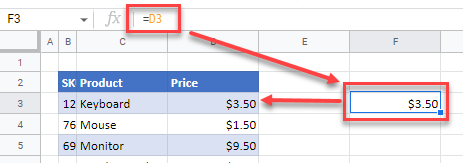# Formula to Copy Value to Another Cell in Excel & Google Sheets

This tutorial demonstrates how to create a formula to copy a value to another cell in Excel and Google Sheets.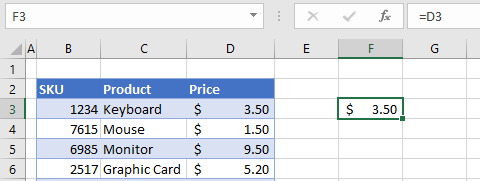## Create Formula to Copy Value

1. Click in the cell where you wish the formula to be created.
2. Press the = (equal) key on the keyboard, and then click on the cell that contains the value you need.
The formula is built for you using a cell reference.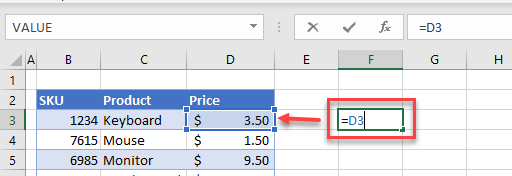``=D3``
1. Press the ENTER key on the keyboard. Your formula appears in the formula bar while the value appears in the actual cell.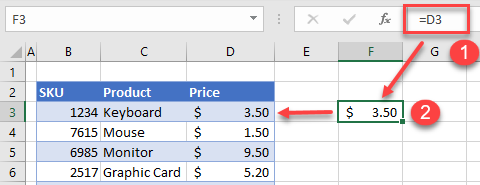1. Change the value in the original cell (e.g., D3) to update the value in the formula.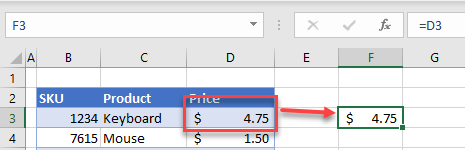## Functions to Return Cell Values

You can also return a value from one cell to another cell by using Excel functions.

The VLOOKUP Function, for example, allows you to look up values in one area of the worksheet, and return the value to another area of the worksheet.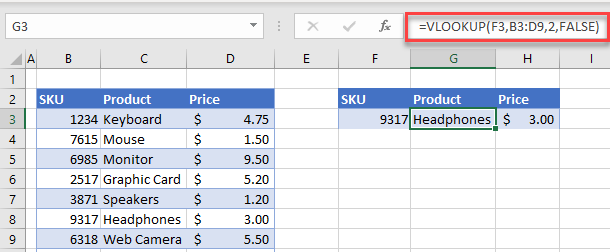You can also use HLOOKUP to return a similar result.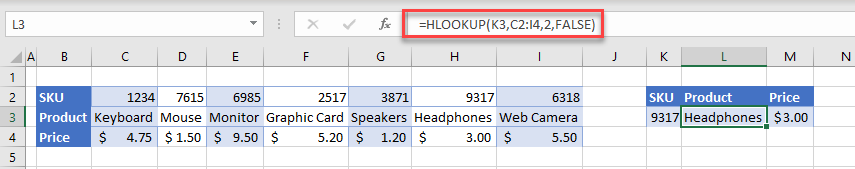Alternatively, you can use the INDEX and MATCH Functions, depending on how the data is set up.## Copy to Another Cell With VBA

You can also use VBA to copy the values using a formula to another cell.

1. In the VBE, create a module within your workbook, and then create the following macro.
``````Sub CopyValueWithFormula()
Range("G3") = "=D3"
End Sub``````
1. Switch back to Excel, and then in the Ribbon, go to Developer > Code > Macros.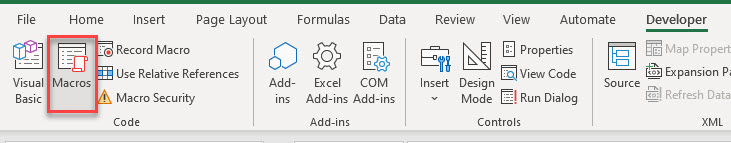1. Select the macro you created and then click Run.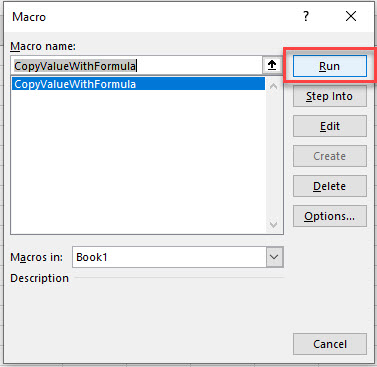1. Cell G3 is populated with the formula:
``=D3``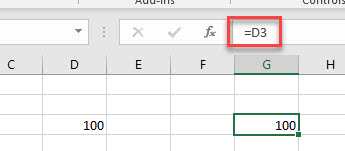## Formula to Copy Value in Google Sheets

Google Sheets works in exactly the same way as Excel when copying a cell value using a formula.